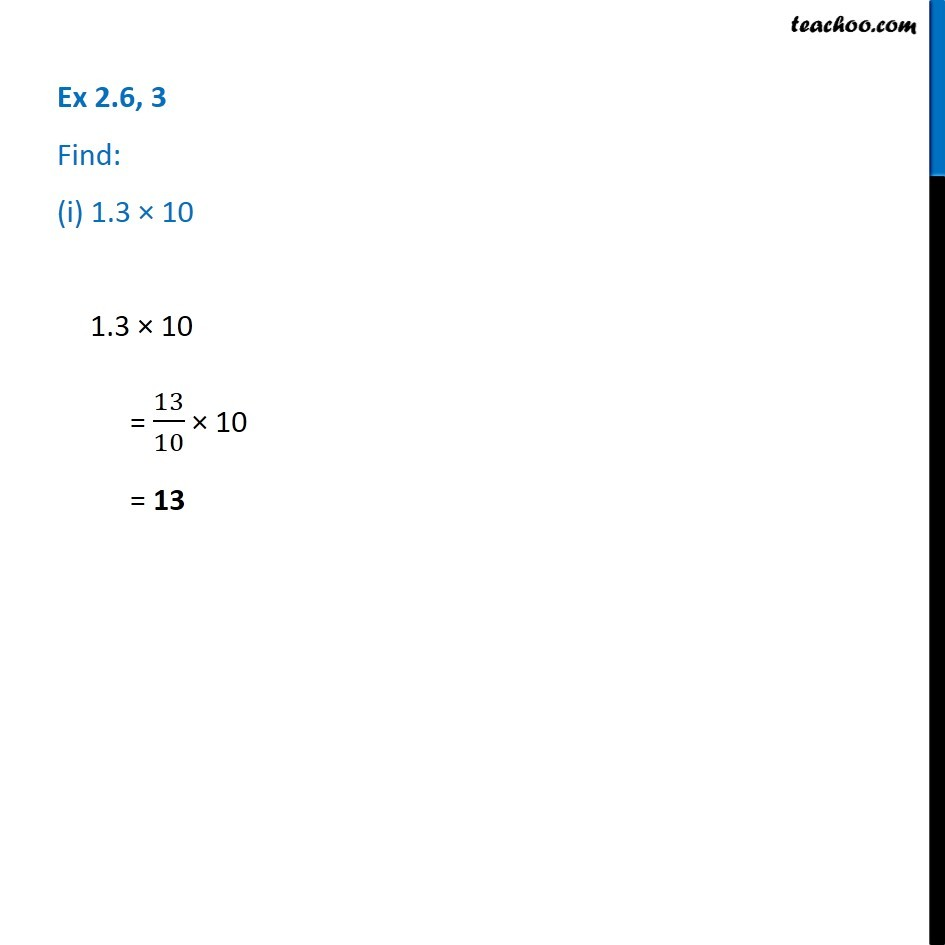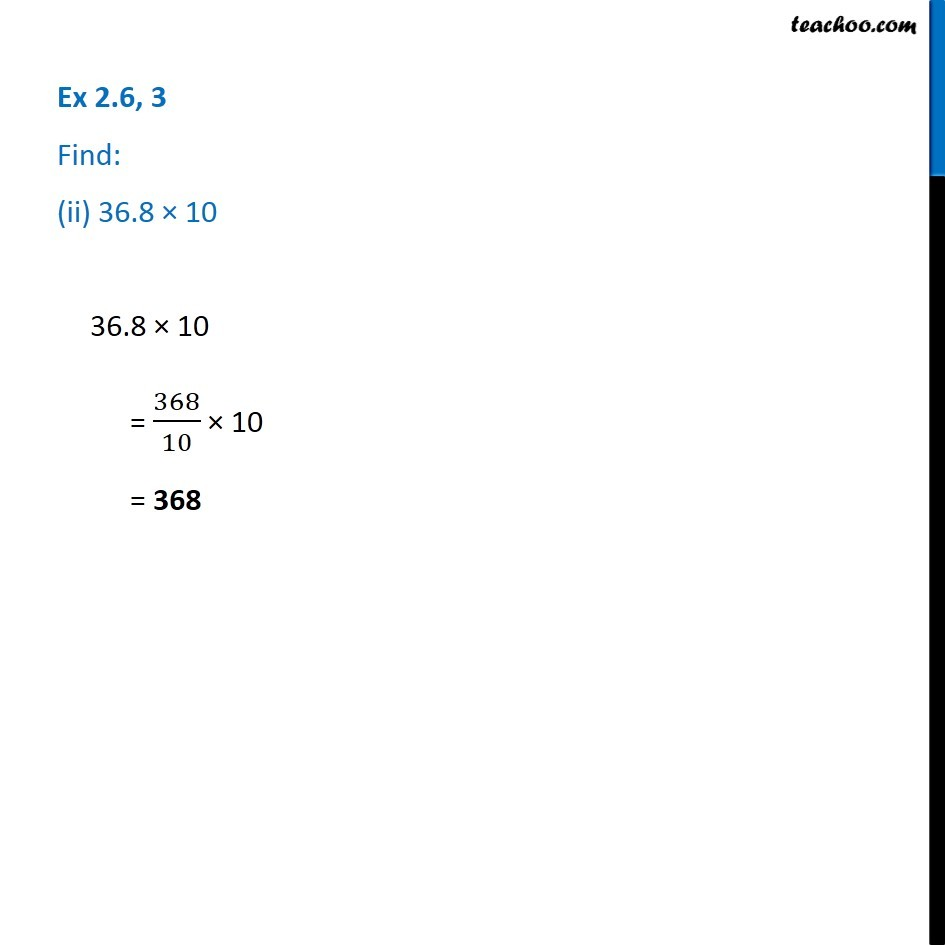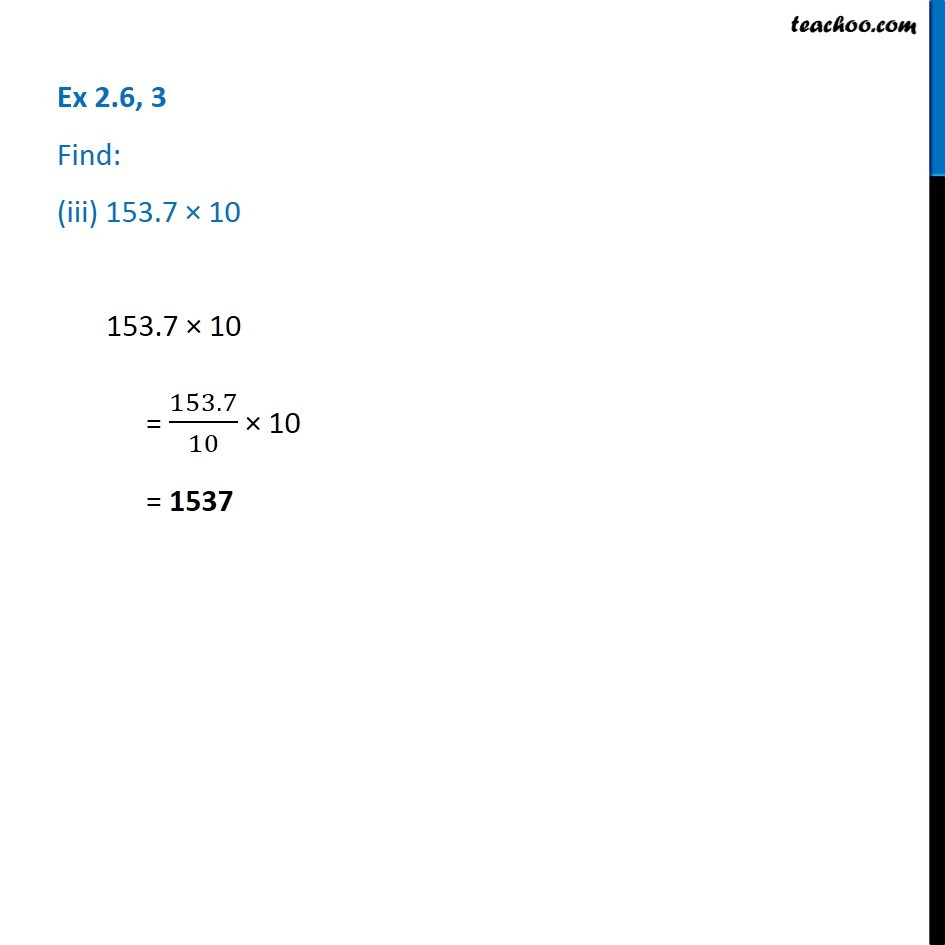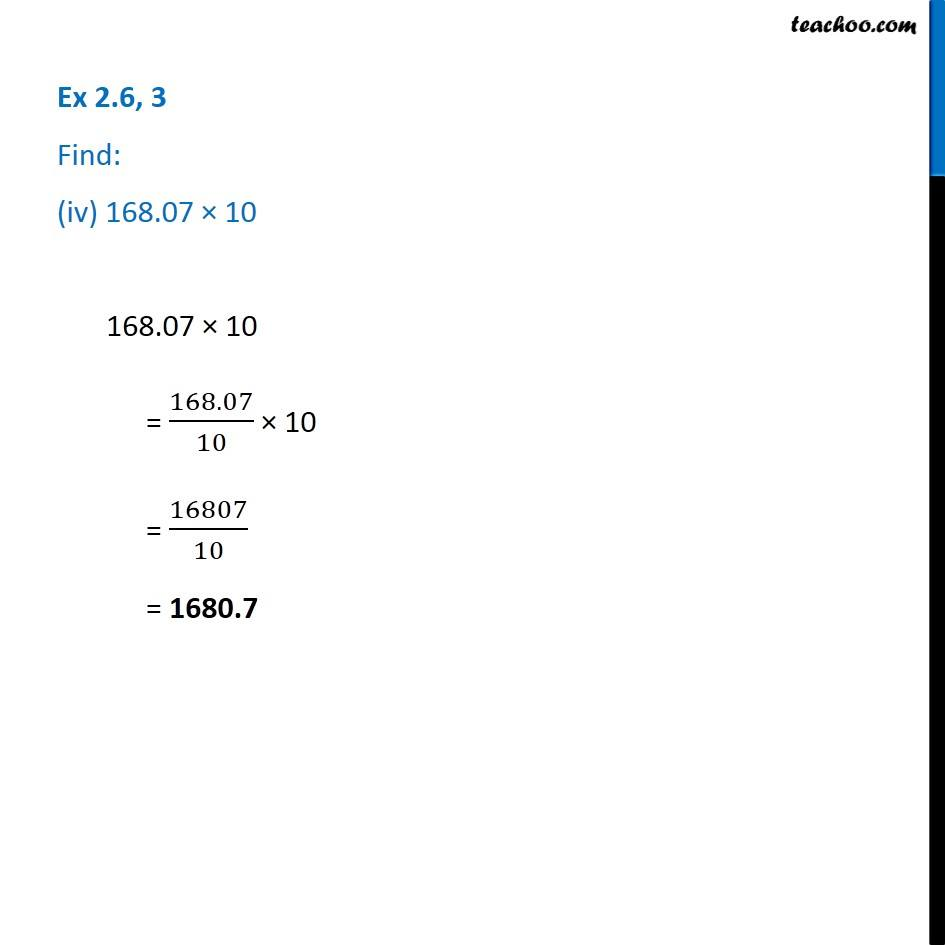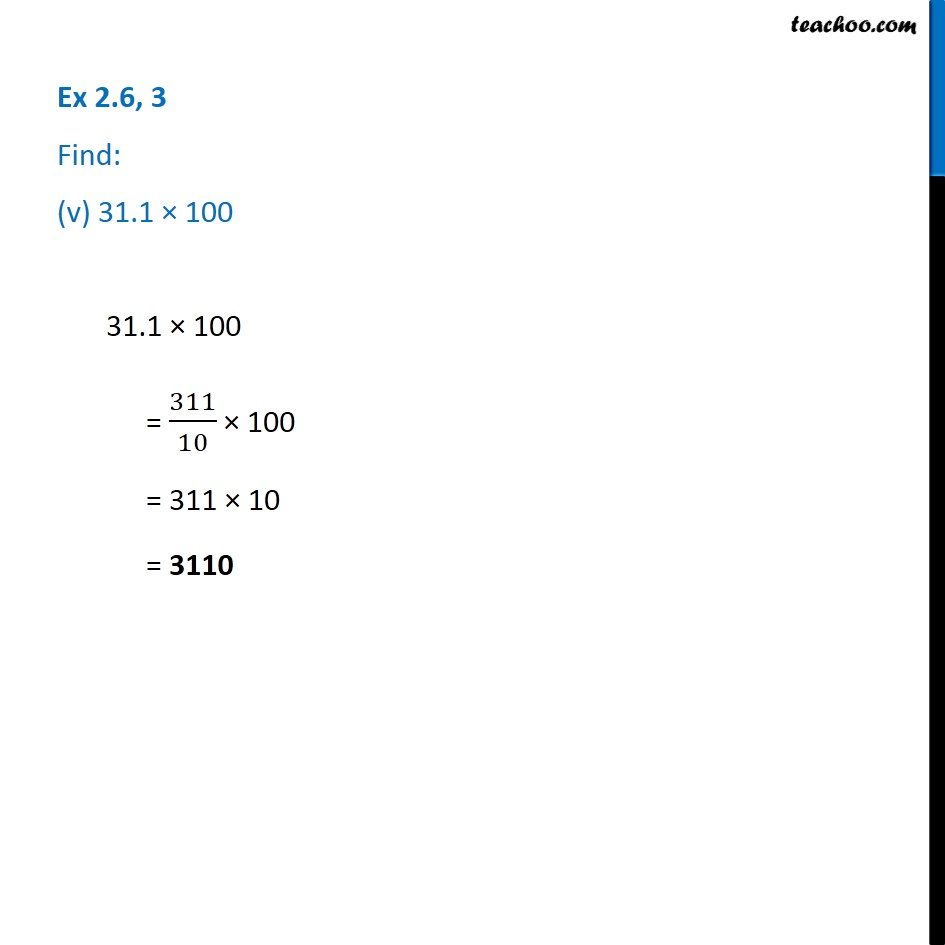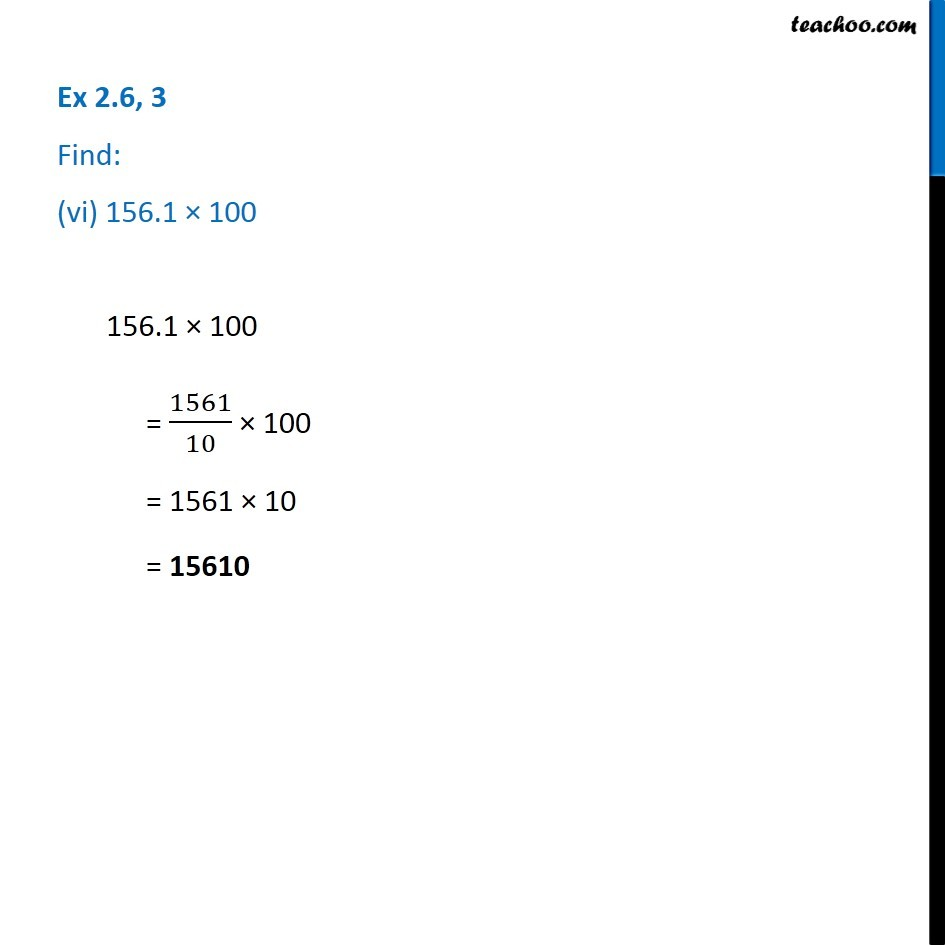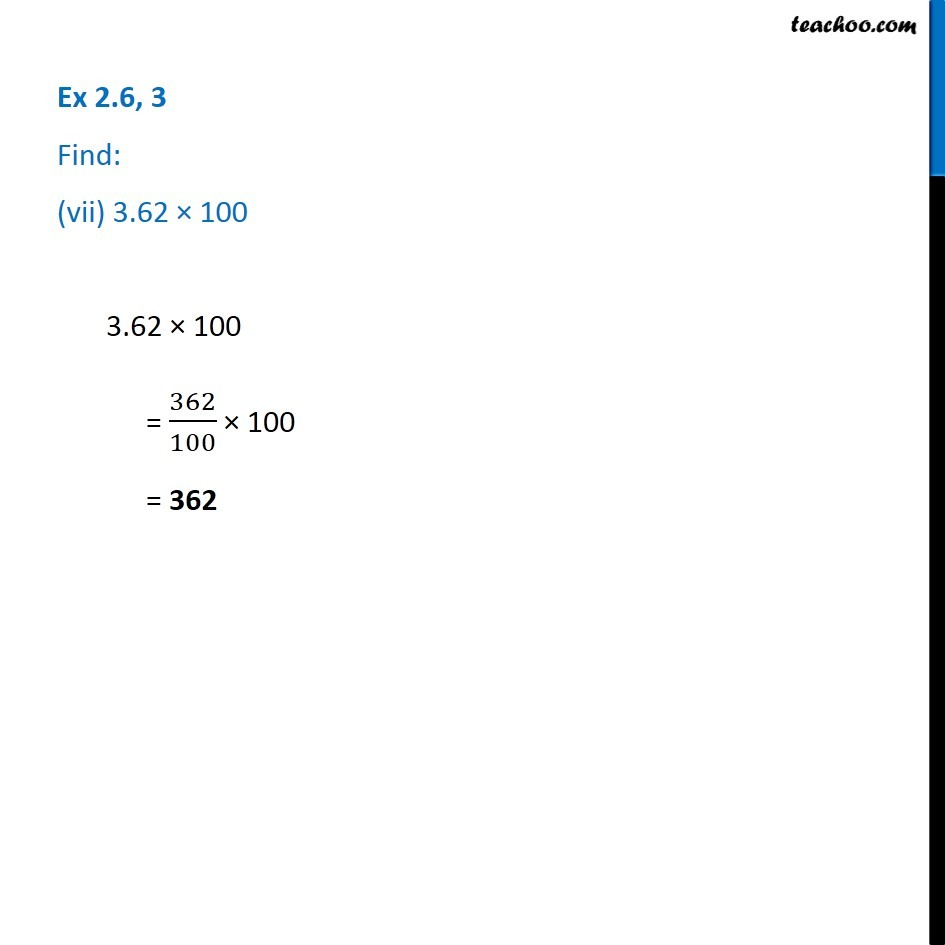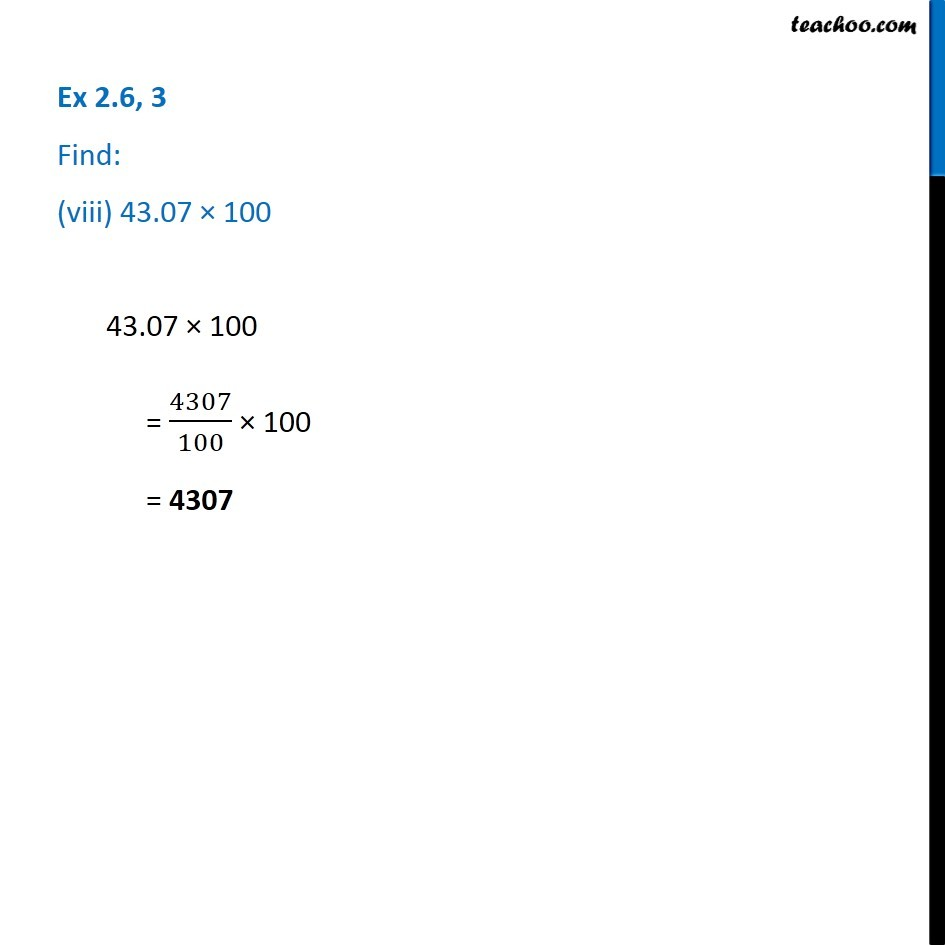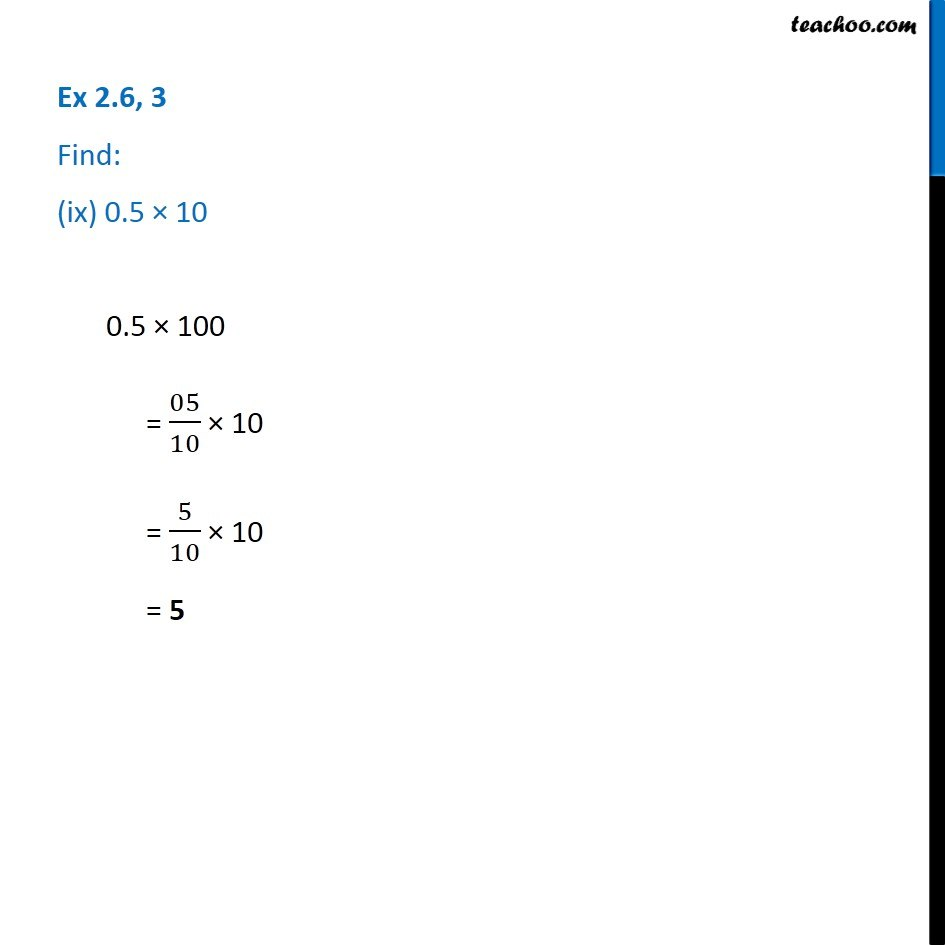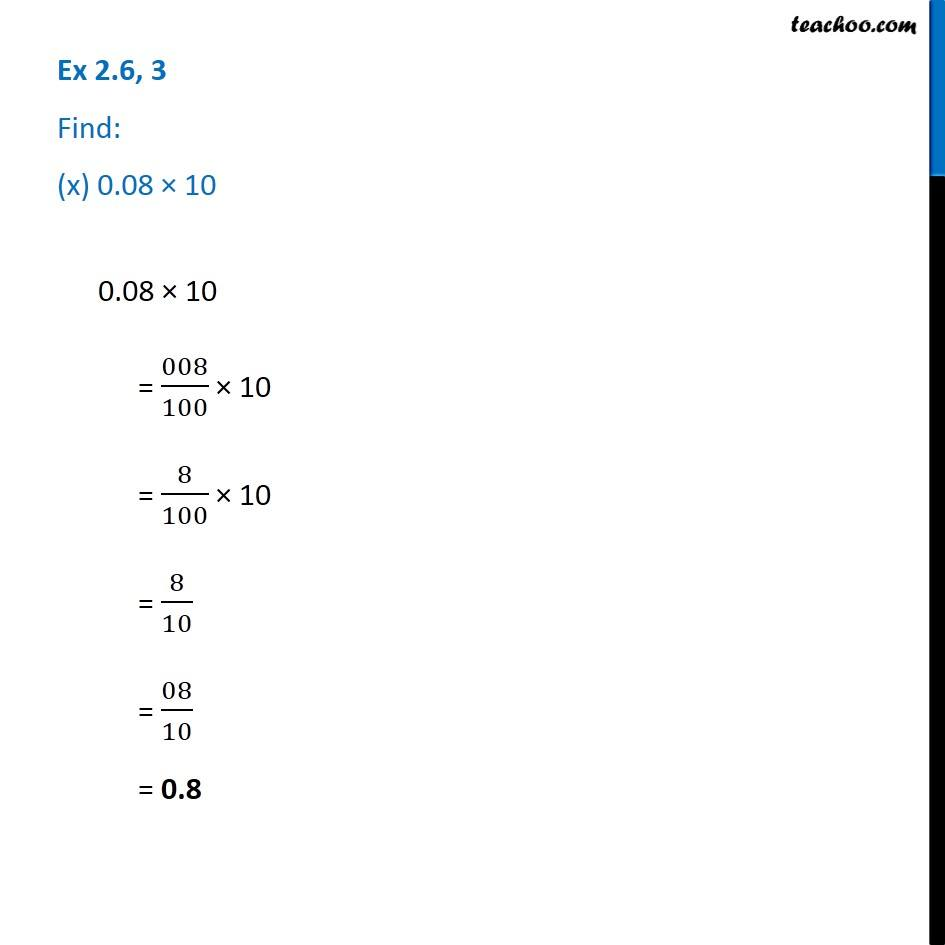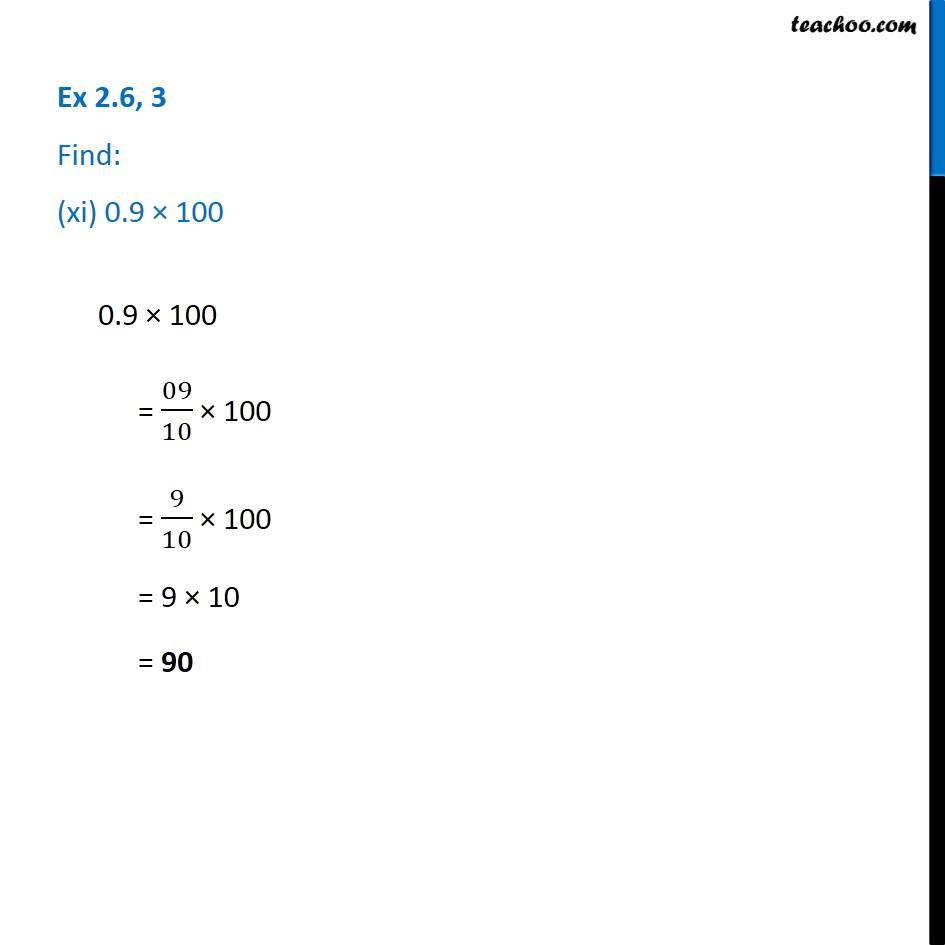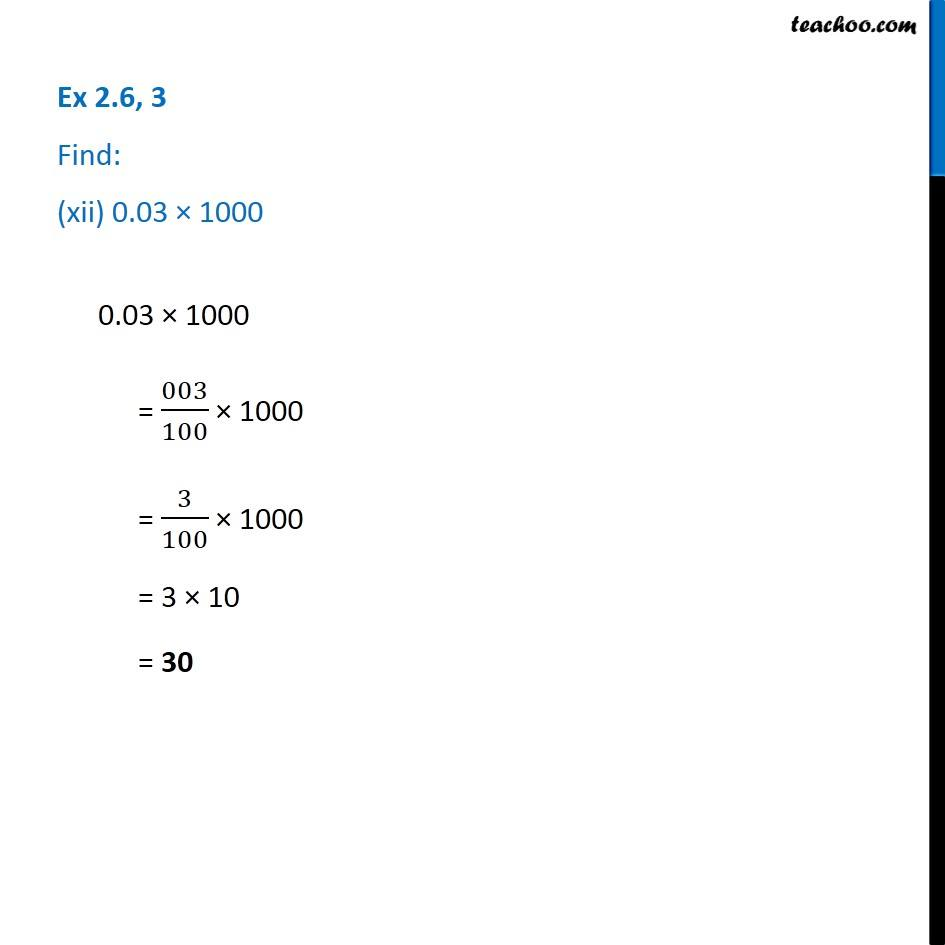1. Chapter 2 Class 7 Fractions and Decimals
2. Concept wise
3. Multiplication of Decimals

Transcript

Ex 2.6, 3 Find: (i) 1.3 × 10 1.3 × 10 = 13/10 × 10 = 13 Ex 2.6, 3 Find: (ii) 36.8 × 1036.8 × 10 = 368/10 × 10 = 368 Ex 2.6, 3 Find: (iii) 153.7 × 10153.7 × 10 = 153.7/10 × 10 = 1537 Ex 2.6, 3 Find: (iv) 168.07 × 10168.07 × 10 = 168.07/10 × 10 = 16807/10 = 1680.7 Ex 2.6, 3 Find: (v) 31.1 × 100 31.1 × 100 = 311/10 × 100 = 311 × 10 = 3110 Ex 2.6, 3 Find: (vi) 156.1 × 100156.1 × 100 = 1561/10 × 100 = 1561 × 10 = 15610 Ex 2.6, 3 Find: (vii) 3.62 × 100 3.62 × 100 = 362/100 × 100 = 362 Ex 2.6, 3 Find: (viii) 43.07 × 10043.07 × 100 = 4307/100 × 100 = 4307 Ex 2.6, 3 Find: (ix) 0.5 × 100.5 × 100 = 05/10 × 10 = 5/10 × 10 = 5 Ex 2.6, 3 Find: (x) 0.08 × 100.08 × 10 = 008/100 × 10 = 8/100 × 10 = 8/10 = 08/10 = 0.8 Ex 2.6, 3 Find: (xi) 0.9 × 1000.9 × 100 = 09/10 × 100 = 9/10 × 100 = 9 × 10 = 90 Ex 2.6, 3 Find: (xii) 0.03 × 10000.03 × 1000 = 003/100 × 1000 = 3/100 × 1000 = 3 × 10 = 30

Multiplication of Decimals